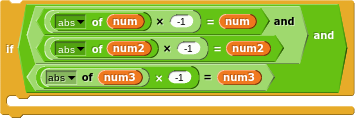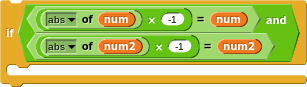# Workarounds for blocks Snap doesn't have (Part 2)

Continuing the discussion from Workarounds for blocks Snap doesn't have (Part 1).

Previous discussions:

Now that we have ≤ and ≥ you should add them to the menu of end tests.

Before we had FOR as a primitive, we implemented it this way:

The cool part is the CALL (END TEST) and ringifying the test function.

we also have ≠

Yes, but ≠ is an unlikely end test in a FOR loop.

oh, ok.

like this?

Sure. But actually you could cut that code in half because each end test makes sense only with one step sign. For example, if you're using i < end as the end test, then the step sign has to be negative, because with a positive step size, either the end test is met right away or it will never be met. So that combination is an error.

so do i cut the i < end

oh, and sorry that i didn't respond for like 2 hours

No, you don't change the program until you really understand what I meant, not just edit it because I said so!

i < end is a good end test if the step size is negative. Think about some examples.

for i = 1 step -1 until i < 5: What will this do? i=1 is already less than 5, so it won't do anything at all.

for i = 5 step -1 until i < 1: What will this do? It'll run the script in the C-slot with i=5, i=4, i=3, i=2, i=1, and then stop when it tries i=0. This is a valid case.

for i = 1 step 1 until i < 5: Again, i=1 is already less than 5, so it won't do anything.

for i = 5 step 1 until i < 1: What about this? i=5 isn't less than 1, so it runs the script with i=5, i=6, i=7, i=8,... forever.

I can't think of any other interesting cases that aren't essentially one of those. I mean, there are cases like for i = 5 step -1 until i < 5, which will run the script exactly once, with i=5. But that's just an edge case, where start=end.

Now go through the four examples above with > as the comparator, and work out which make sense and which don't.

When you understand all that, then you can think about modifying your code.

You don't need to be sorry about something like that. This is a forum, not an instant messaging service.

i guesswill help in the i < end

What's important is comparing i with end, not comparing either of them with zero. (That's what you're doing by looking at the sign of the number.)it was just something to detect if the number is negative so the i < end would work

Whether i < end will work has nothing to do with whether either number is negative.

for i = -10 to -5 needs i > end as its end test.

for i = -5 to -10 needs i < end as its end test.

Umm. I hate to ruin your mood, but... You have the following (mixed with other tests) near the beginning of that block:

set (i) to (num)
if (num) > (num2)
repeat until (i) > (num2)
...


What's that repeat until going to do? We've already determined that num > num2, and i=num, so i is already bigger than num2 before the repeat until. So it's never going to do anything. Right?

I have one question, How to post blocks?

Thanks!

right click the block you want to take a picture of, click script pic and then upload the image